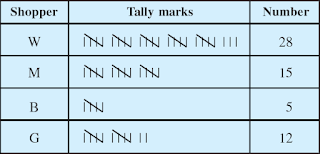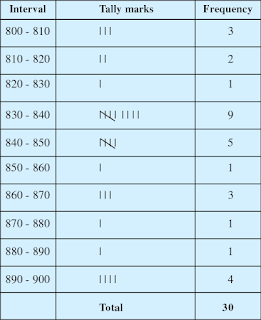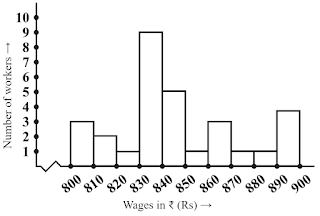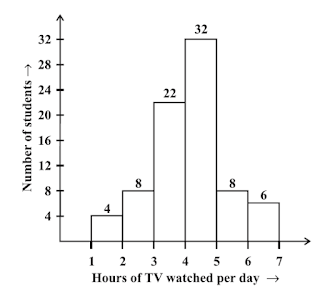# vs.eyeandcontacts.com

## Chapter 5 Data Handling Exercise 5.1

Question 1: For which of these would you use a histogram to show the data:
a) The number of letters for different areas in a postman’s bag.
b) The height of competitors in an athletics meet.
c) The number cassettes produced by 5 companies.
d) The number of passengers boarding trains from 7.00 a.m. to 7.00 p.m. at a station. Give reason for each.
A histogram is a graphical representation of a grouped frequency distribution with continuous classes.
The cases mentioned in options (b) and (d) can be divided into class intervals, histogram can be used to show the data.
The cases mentioned in options (a) and (c) cannot be divided into class intervals, histogram cannot be used to represent the data.

Question 2: The shoppers who come to a departmental store are marked as: man (M), woman (W), boy (B) or girl (G). The following list gives the shoppers who came during the first hour in the morning.
W W W G B W W M G G M M W W W W G B M W B G G M W W M M W W W M W B W G M W W W W G W M M W M W G W M G W M M B G G W.
Make a frequency distribution table using tally marks. Draw a bar graph to illustrate it.

Frequency distribution table:Bar-graph:Question 3: The weekly wages (in ₹) of 30 workers in a factory are:
830, 835, 890, 810, 835, 836, 869, 845, 898, 890, 820, 860, 832, 833, 855, 845, 804, 808, 812, 840, 885, 835, 835, 836, 878, 840, 868, 890, 806, 840.
Using tally marks, make a frequency table with intervals as 800 - 810, 810 - 820 and so on.
The frequency table with intervals as 800 - 810, 810 - 820 and so on, using tally marks is given below:Question 4: Draw a histogram for the frequency table made for the data in Question 3 and answer the following questions.
(i) Which group has the maximum number of workers?
(ii) How many workers earn ₹ 850 and more?
(iii) How many workers earn less than ₹ 850?

i) 830-840 is the group having maximum number of workers.
ii) 10 workers are earning ₹850 and more.
iii) 20 workers are earning less than ₹850.

Question 5: The number of hours for which students of a particular class watched television during holidays is shown through the given graph.
(i) For how many hours did the maximum number of students watch T.V.?
(ii) How many students watched TV for less than 4 hours?
(iii) How many students spent more than 5 hours in watching TV?